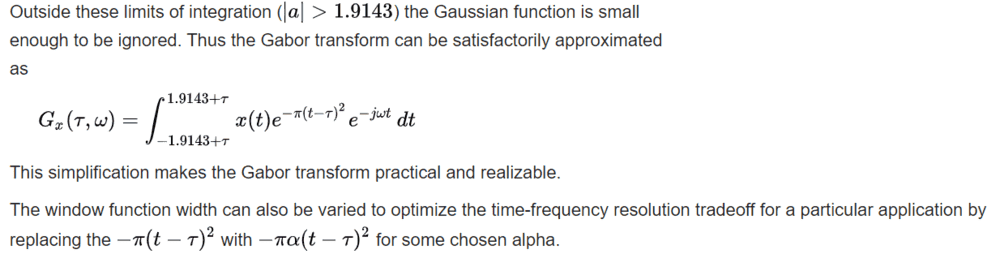# Calculating 1D spectrum from 2D spectrum

nkinar
Hello---

I am reading a paper which presents a method to determine attenuation (and Q factors) from reflection seismic data (Y. Wang, "Q analysis on reflection seismic data," Geophysical Research Letters, Vol. 31, 2004).

To perform signal processing on a seismic trace, the paper describes the following procedure:

(1) From the real and complex parts of the Gabor spectrum transform, compute the (real numbered) amplitude spectrum $$U(t, \omega)$$ on a seismic trace $$s(t)$$, where $$t$$ is the time (s), $$\omega$$ is the angular frequency (1/s), and $$\omega = 2 \pi f$$, where $$f$$ is the frequency in Hz.

(2) Define $$\chi = t \omega$$ as the product of $$t$$ and $$\omega$$, and transform the 2D spectrum $$U(t, \omega)$$ into the 1D spectrum $$U(t\omega) = U(\chi)$$.

The paper does not describe how to transform $$U(t, \omega)$$ into $$U(\chi)$$.

Would numerical integration be able to do this transformation? How might I proceed?

## Answers and Replies

Mentor
2021 Award
For the sake of completeness (https://en.wikipedia.org/wiki/Gabor_transform):In our case we have
$$U_x(\tau \, , \,\omega)=\displaystyle{\int_{-1.9143+\tau}^{1.9143+\tau}}x(t)e^{-\pi(t-\tau)^2}e^{-i\omega t}\,dt )$$
and I'm a bit confused whether ##t## or ##\tau## is meant, i.e. if we are talking about a simple variable substitution. I assume that the exponent ##-\omega t## is abbreviated by ##\chi## and we have such a substitution of the integral variable. The real case applications of the Gabor transform lives with approximations. So maybe the ##\alpha## in the Wikipedia article can be used to simplify the other terms of the integrand. Or - what could be as well - ##U(\chi)## is a sloppy notation for ##U(\tau,\chi)##.

nkinar
Thanks, I think that you are right about the notation and the substitution.